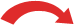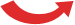### There are 3 simple steps to subtract fractions

• Step 1. Make sure the bottom numbers (the denominators) are the same
• Step 2. Subtract the top numbers (the numerators). Put the answer over the same denominator.
• Step 3. Simplify the fraction (if needed).

### Example 1:

3 4 − 1 4

Step 1. The bottom numbers are already the same. Go straight to step 2.

Step 2. Subtract the top numbers and put the answer over the same denominator:

3 4 − 1 4   =   3 − 1 4   =   2 4

Step 3
. Simplify the fraction:

2 4 = 1 2

(If you are unsure of the last step see Equivalent Fractions.)

### Example 2:

1 2 − 1 6

Step 1. The bottom numbers are different. See how the slices are different sizes? We need to make them the same before we can continue, because we can’t subtract them like this:

 1 2 − 1 6 = ?To make the bottom numbers the same, multiply the top and bottom of the first fraction (1/2) by 3 like this:

 × 3× 3

And now our question looks like this:

The bottom numbers (the denominators) are the same, so we can go to step 2.

Step 2. Subtract the top numbers and put the answer over the same denominator:

3 6 − 1 6   =   3 − 1 6   =   2 6

In picture form it looks like this:

 3 6 − 1 6 = 2 6Step 3. Simplify the fraction:

2 6 = 1 3

### With Pen and Paper

And here is how to do it with a pen and paper (press the play button):

## Subtracting Mixed Fractions

I have a special page on Adding and Subtracting Mixed Fractions.

## Making the Denominators the Same

In the previous example it was easy to make the denominators the same, but it can be harder … so you may need to use either the

### Example: Cupcakes

You want to sell cupcakes at a market:

• You get paid 2 5 of total sales
• But you have to pay 1 4 of total sales for the stall

How much do you get?

We need to subtract 1 4 from 2 5

2 5 − 1 4   =   ? ?

First make the bottom numbers (the denominators) the same.

Multiply top and bottom of 2/5 by 4:

2 × 4 5 × 4 − 1 4   =   ? ?

And multiply top and bottom of 1/4 by 5:

2 × 4 5 × 4 − 1 × 5 4 × 5   =   ? ?

Now do the calculations:

8 20 − 5 20   =   8 − 5 20   =   3 20

Answer: you get to keep 3 20 of total sales.